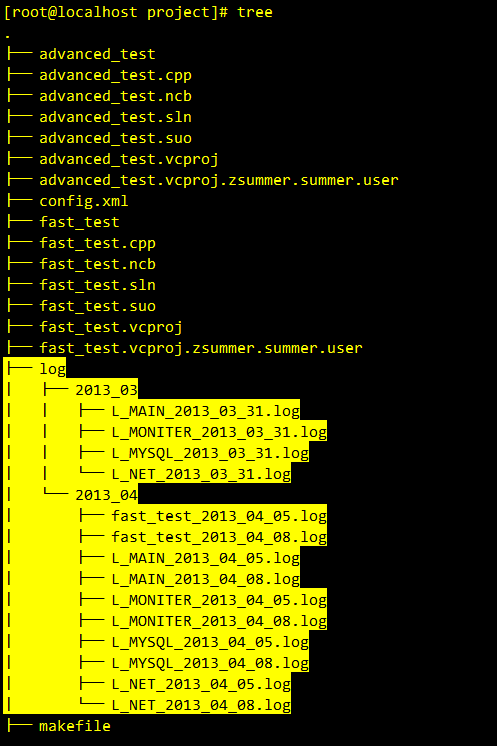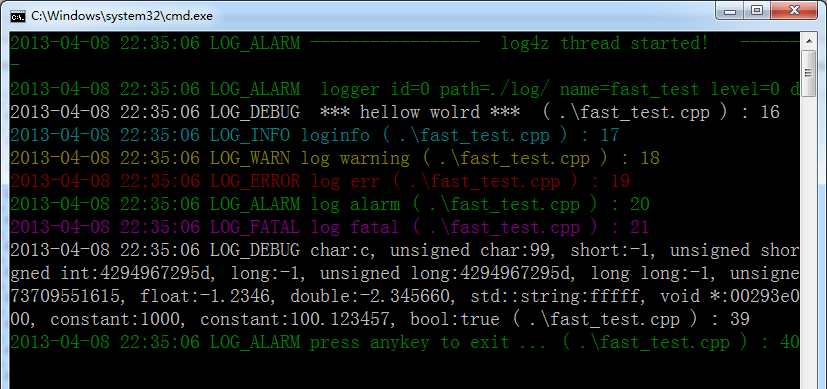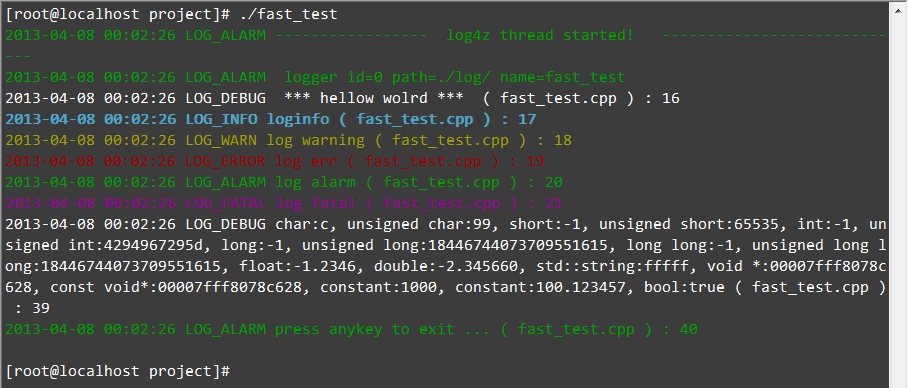• Articles
• log4z v1.2.0 stable version publish
Apr 7, 2013 (last update: Apr 14, 2013)

log4z v1.2.0 stable version publishintro

Log4z is an open source C++ lightweight log library. It provides in a C++ application log and trace debug function. Using log4z can be very easy and convenient to debug log or track for conveying information to the screen, the log file

the open source site:

https://github.com/zsummer/log4z
tags:

https://github.com/zsummer/log4z/tags

1. MIT open source license,very liberal.
2. lightweight and cross platform.
3. complete priority level control.
4. muilti log output, thread safe.
5. complete file's configure.
6. display to screen with different color.

fast_test.cpp : the simple demo

 123456789101112131415161718192021222324252627282930313233343536373839404142 #include "../log4z.h" #include #include using namespace zsummer::log4z; int main(int argc, char *argv[]) { //start log4z ILog4zManager::GetInstance()->Start(); //LOGD: LOG WITH level LOG_DEBUG //LOGI: LOG WITH level LOG_INFO //... LOGD(" *** " << "hellow wolrd" <<" *** "); LOGI("loginfo"); LOGW("log warning"); LOGE("log err"); LOGA("log alarm"); LOGF("log fatal"); LOGD("char:" <<'c' << ", unsigned char:" << (unsigned char) 'c' << ", short:" << (short) -1 << ", unsigned short:" << (unsigned short) -1 << ", int:" << (int) -1 << ", unsigned int:" << (unsigned int) -1 << ", long:" << (long) -1 << ", unsigned long:" << (unsigned long) -1 << ", long long:" << (long long) -1 << ", unsigned long long:" << (unsigned long long) -1 << ", float:" << (float) -1.234567 << ", double:" << (double) -2.34566 << ", std::string:" << std::string("fffff") << ", void *:" << ( int *) argv << ", const void*:" << (const int *) argv << ", constant:" << 1000 << ", constant:" << 100.12345678 << ", bool:" << (bool) true); LOGA("press anykey to exit ..."); getchar(); return 0; }

compile

 in VS join the log4z.h and log4z.cpp in linux, need append -lpthread. example: g++ -lpthread -o test main.cpp ../log4z.cpp

the screenshot

log4z_log_tree:log4z_windows_fast_output:log4z_linux_fast_output